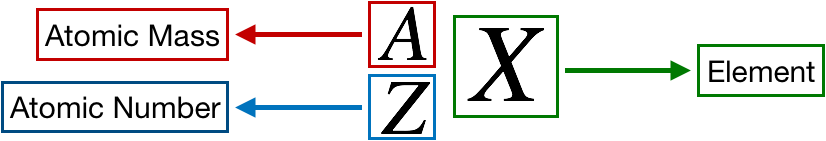# Problem: Fill in the gaps in the following table:SymbolCo3+Protons347680Neutrons46116120Electrons3678Mass number59Net charge2+Complete the second row of the table.

###### FREE Expert Solution

We’re being asked to complete the second row of the table given the number of protons or atomic number.

Recall that the symbol for an element or ion is as follows:Recall that:

• Atomic Mass = A number of protons + number of neutrons
• Atomic NumberZ = number of protons = number of electrons

From the periodic table, we can find the atomic number of cobalt (Co):

92% (456 ratings)###### Problem Details
Fill in the gaps in the following table:

 Symbol Co3+ Protons 34 76 80 Neutrons 46 116 120 Electrons 36 78 Mass number 59 Net charge 2+

Complete the second row of the table.